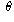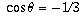Navigation Panel:(These buttons explained below)Question Corner and Discussion Area

# Angle Between Vertices of a Tetrahedron

Asked by Lee Lude, student, Michigan City High School on February 5, 1998:
Given a regular tetrahedral with a point in the center, find the angle formed from this center point to two corners (next to each other) in the tetrahedral. In chemistry terms you are actually proving that the the electrons in a tetrahedral shaped molecule are 109.5 degrees apart. No where in the proof however can you use the 109.5 degrees. This is the angle that you want to find.
This souds like an assignment question. Our goal here is to help you understand mathematical concepts (especially ones that go beyond the standard curriculum), so we would prefer you to ask "Here is a question I have been given, and I would like to understand better how I should go apart tackling such-and-such a part of it . . . " rather than simply asking us the same question that was asked of you.

There are several ways to tackle this question. The simplest involves using vectors. Think about the four vectors from the centre of the tetrahedron to the four corners. You know several things about these vectors:

1. These vectors all have the same length l.
2. The anglebetween any pair of vectors is the same, and from the theory of dot products one has the fact that(where u and v are any two of the vectors).
3. By symmetry, the vector sum of all four vectors is zero.
Combining these pieces of information, if u, v, w, and x are the four vectors, you haveand therefore, which you can solve forto get an answer of approximately 109.5 degrees.

[ Submit Your Own Question ] [ Create a Discussion Topic ]

This part of the site maintained by (No Current Maintainers)
Last updated: April 19, 1999
Original Web Site Creator / Mathematical Content Developer: Philip Spencer
Current Network Coordinator and Contact Person: Joel Chan - mathnet@math.toronto.edu

Navigation Panel: